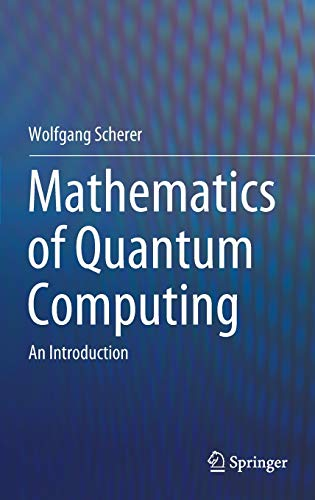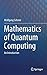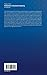Sunday , January 24th 2021

# Mathematics of Quantum Computing: An Introduction#### Product Features:

This textbook presents the elementary aspects of quantum computing in a mathematical form. It is intended as core or supplementary reading for physicists, mathematicians, and computer scientists taking a first course on quantum computing. It starts by introducing the basic mathematics required for quantum mechanics, and then goes on to present,…
Price as on: 2020-01-17 15:05:27

#### Product Description

This textbook presents the elementary aspects of quantum computing in a mathematical form. It is intended as core or supplementary reading for physicists, mathematicians, and computer scientists taking a first course on quantum computing. It starts by introducing the basic mathematics required for quantum mechanics, and then goes on to present, in detail, the notions of quantum mechanics, entanglement, quantum gates, and quantum algorithms, of which Shor’s factorisation and Grover’s search algorithm are discussed extensively. In addition, the algorithms for the Abelian Hidden Subgroup  and Discrete Logarithm problems are presented and the latter is used to show how the Bitcoin digital signature may be compromised. It also addresses the problem of error correction as well as giving a detailed exposition of adiabatic quantum computing. The book contains around 140 exercises for the student, covering all of the topics treated, together with an appendix of solutions.

## Complexity Theory, Game Theory, and Economics: The

This monograph comprises a series of ten lectures divided into two parts. Part 1, referred to as the Solar…

## Computer Science Education

This book provides an overview of how to approach computer science education research from a pragmatic perspective. It represents…

## Supervised Learning with Quantum Computers (Quantum

Quantum machine learning investigates how quantum computers can be used for data-driven prediction and decision making. The books summarises…